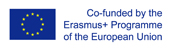This project has been funded with support from the European Commission.
This web site reflects the views only of the author, and the Commission cannot be held responsible for any use which may be made of the information contained therein.

Select language   >   IT EN RO PL LT

### Probability Practice Problems

Subject taught
- Math

Type of Product
- Multiple choice questionnaire
- Videos
- Web Site/Portal

Language Skills Developed
- Interaction
- Listening
- Speaking
- Writing

Transferable/Scientific Skills Developed
Students will learn to calculate the possibilities of probabilities. Students will be able to list the possible probabilities of visas as well as count the many probabilities they satisfy by submitting a condition.

Description
In this lesson, students will learn how to solve probability problems and develop analytical skills.
General aims: The lesson aims to advance students‘ problem-solving skills by involving them in solving real-life problems.
Linguistic aims: Students will know the basic vocabulary in English connected to Probability and Mathematics, develop reading skills.
Subject-specific aims: Students will be able to identify all possible probability variations of the given task. Students will be able to calculate all probable and not probable occurrences of the same task. Also, students will gain confidence in their Math skills.
Target group age: This lesson is suitable for 15-16 years old, students who study in year 9 and year 10. This material can be used by anyone who wants to learn mathematical operations with probability.
Level of competence in English (CEFR) B1/B1+
Time required to use the resource with the students: The material can be done in 90 minutes with the possibility to expand if a teacher wants to do that. How to use it: On this website, students can find exercises and examples of probability, also find ways and explanations on how to solve a certain task. All tasks thoroughly explained in great detail, step-by-step explanations on understanding the concept of probability. In this lesson, the teacher can present some new vocabulary (related to Maths) for students in English and give translations into their native language. Students together with the teacher, do the basic examples at the same time revising geometrical figures, months, news in the world, such as sports, tennis players. At the end of the lesson, the teacher can give out exit slips to students, where they should answer basic questions about the lesson and probability, such as: provide a possible solution to a given probability problem?